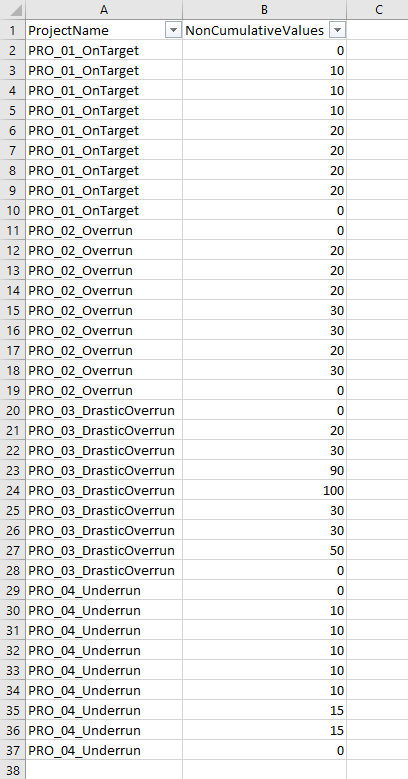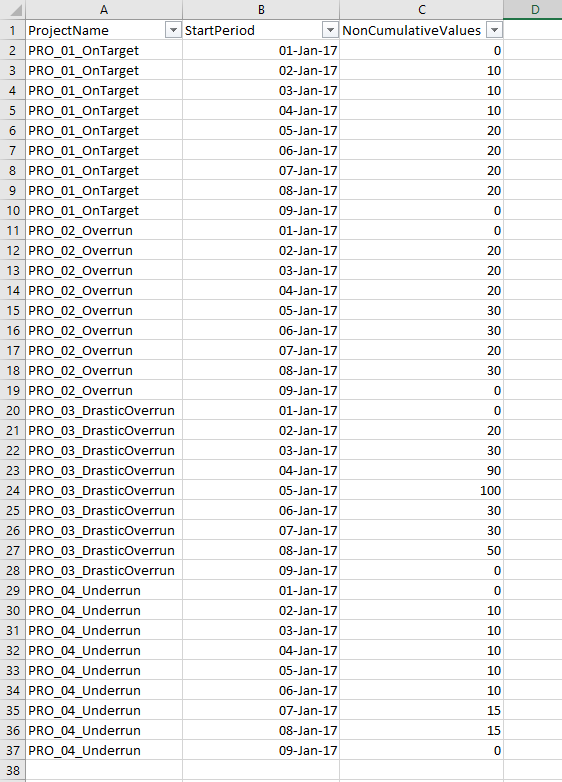cancel
Showing results for
Did you mean:Helper I

## Cumulative column after name filter

Hello,

I would like to create a column that calculates cumulative values after filtering the ProjectName column.So for example, if I filter the ProjectName column and choose PRO_01_OnTraget, then the result should be like that:I would appreciate your assistance so much.

Best regards,

Hayman

1 ACCEPTED SOLUTIONHelper I

I found how it should be:

Since the filter is based on a Text value (not date nor numerical), then filter using the "Values" function

So it should be:

=CALCULATE(SUM('Table1'[NonCumulativeValues] ), FILTER ( ALL ( 'Table1' ), [StartPeriod] <= MAX ( [StartPeriod] )), VALUES ( 'Table1'[ProjectName] ) ) )

Best regards,

Hayman

5 REPLIES 5Microsoft

What determines the order?  Do you have date column that can be used?

Proud to be a Datanaut!Helper I

Dear @Phil_Seamark

I can have a date column that will look like thatRegards,

HaymanMicrosoft

Give this measure a crack.  I think it might be close

```Measure =
CALCULATE (
SUM ( 'Table1'[NonCumulativeValues] ),
FILTER (
ALL ( 'Table1' ),
[StartPeriod] <= MAX ( [StartPeriod] )
&& Table1[ProjectName] = MAX ( 'Table1'[ProjectName] )
)
)```

Proud to be a Datanaut!Helper I

I get an error:

The function MAX takes an argument that evaluates to numbers or dates and cannot work with values of type String

I think this is coming from the last MAX function in the measure, since the "ProjectName" is a string.

Regards,

HaymanHelper I

I found how it should be:

Since the filter is based on a Text value (not date nor numerical), then filter using the "Values" function

So it should be:

=CALCULATE(SUM('Table1'[NonCumulativeValues] ), FILTER ( ALL ( 'Table1' ), [StartPeriod] <= MAX ( [StartPeriod] )), VALUES ( 'Table1'[ProjectName] ) ) )

Best regards,

HaymanAnnouncements#### Exclusive opportunity for Women!

Join us for a free, hands-on Microsoft workshop led by women trainers for women where you will learn how to build a Dashboard in a Day!#### Power Platform Conference-Power BI and Fabric Sessions

Join us Oct 1 - 6 in Las Vegas for the Microsoft Power Platform Conference.Top Solution Authors
Top Kudoed Authors
Users online (3,558)